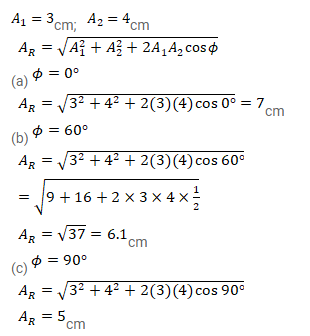# A particle is subjected to two simple harmonic motions of same time

Question:

A particle is subjected to two simple harmonic motions of same time period in the same direction. The amplitude of the first motion is $3.0 \mathrm{~cm}$ and that of the second is $4.0 \mathrm{~cm}$. Find the resultant amplitude if the phase difference between the motions is

(a) $0^{\circ}$,

(b) $60^{\circ}$,

(c) $90^{\circ}$.

Solution: